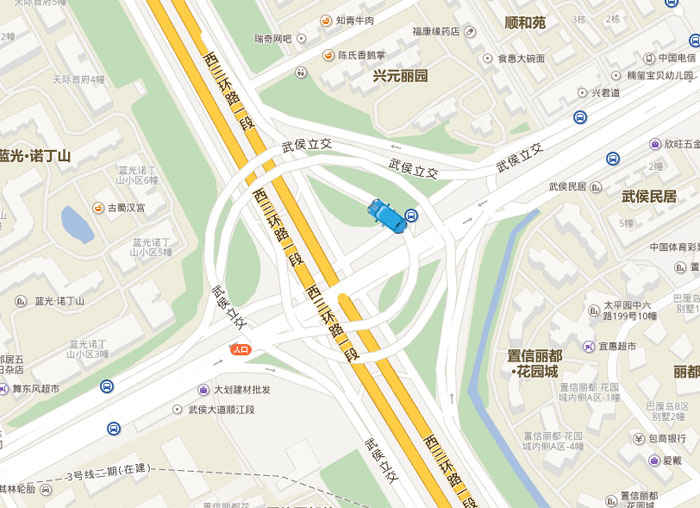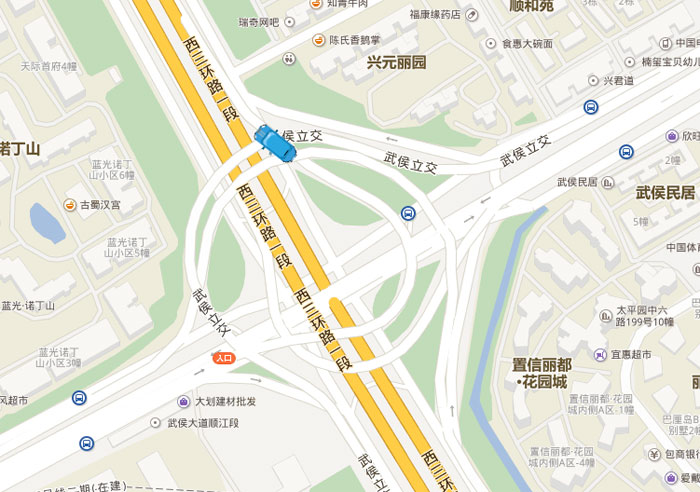# 一、覆盖物在获取坐标数据的同时

`{BMap.Pixel}= map.getMapType().getProjection().lngLatToPoint(BMap.Point);`

``````/*
*缓动效果
*初始坐标，目标坐标，当前的步长，总的步长
*@param{BMap.Pixel} initPos 初始平面坐标
*@parm{BMap.Pixel} targetPos 目标平面坐标
*@param{number} 当前帧数
*@param {number} count 总帧数
*/
this.linear = function (initPos, targetPos, currentCount, count) {
var b = initPos, c = targetPos - initPos, t = currentCount,
d = count;
return c * t / d + b;
}
var x = effect(_prvePoint.x, _newPoint.x, currentCount, count),
y = effect(_prvePoint.y, _newPoint.y, currentCount, count);
``````

`var pos = map.getMapType().getProjection().pointToLngLat(new BMap.Pixel(x, y));`

`me._em._newPointMark.setPosition(pos);`

``````/**
*小车移动
*@param {Point} prvePoint 开始坐标(PrvePoint)
*@param {Point} newPoint 目标点坐标
*@param {Function} 动画效果
*@return 无返回值
*/
this.Move = function (prvePoint, newPoint, effect, setRotation) {
var me = this,
//当前帧数
currentCount = 0,
//初始坐标
_prvePoint = me._projection.lngLatToPoint(prvePoint),//将球面坐标转换为平面坐标
//获取结束点的(x,y)坐标
_newPoint = me._projection.lngLatToPoint(newPoint),
//两点之间要循环定位的次数
count = me._runTime / me._intervalTimer;
//两点之间匀速移动
me._intervalFlag = setInterval(function () {
//两点之间当前帧数大于总帧数的时候，则说明已经完成移动
if (currentCount >= count) {
clearInterval(me._intervalFlag);
} else {
//动画移动
currentCount++;//计数
var x = effect(_prvePoint.x, _newPoint.x, currentCount, count),
y = effect(_prvePoint.y, _newPoint.y, currentCount, count);
//根据平面坐标转化为球面坐标
var pos = map.getMapType().getProjection().pointToLngLat(new BMap.Pixel(x, y));
//设置marker角度(两点之间的距离车的角度保持一致)
if (currentCount == 1) {
//转换角度                     setRotation(prvePoint,newPoint, me._em);
}
//正在移动
me._em._newPointMark.setPosition(pos);
}
}, me._intervalTimer);
me._em._prvePoint = newPoint;
}
``````

me._intervalTimer; 决定了在这两个点之间要走多少个小碎步~~

1，通过控制覆盖物移动数据控制动画效果。这种方法就需要通过

2，通过控制平缓移动过程的总时间与每次执行间隔时间来控制动画效果。此方法就是文中的示例，直接二者做商即可得到要执行的次数。（这种方法适合做实时定位使用，因为下一次定位数据是间隔多少时间后接收，这个使我们可以控制的，所以这个平缓移动的动画过程的总时间也是可以由我们自己控制的）

``````/**
*在每个点的真实步骤中设置小车转动的角度
*@param{BMap.Point} curPos 起点
*@param{BMap.Point} targetPos 终点
*/
this.setRotation = function (curPos, targetPos, em)
{
var me = this;
var deg = 0;
curPos = map.pointToPixel(curPos);
targetPos = map.pointToPixel(targetPos);
if (targetPos.x != curPos.x) {
var tan = (targetPos.y - curPos.y) / (targetPos.x - curPos.x),
atan = Math.atan(tan);
deg = atan * 360 / (2 * Math.PI);
if (targetPos.x < curPos.x) {
deg = -deg + 90 + 90;
} else {
deg = -deg;
}
em._newPointMark.setRotation(-deg);
} else {
var disy = targetPos.y - curPos.y;
var bias = 0;
if (disy > 0)
bias = -1
else
bias = 1
em._newPointMark.setRotation(-bias * 90);
}
return;
}
``````#### 您可能感兴趣的文章:

• nodejs根据ip数组在百度地图中进行定位
• 在网页中插入百度地图的步骤详解
• AngularJS
与百度地图的结合实例
• 百度地图api应用标注地理位置信息(js版)
• android实现百度地图自定义弹出窗口功能
• js
调用百度地图api并在地图上进行打点添加标注
• 百度地图API应用之获取用户的具体位置
• Android下如何使用百度地图sdk
• 百度地图API之本地搜索与范围搜索
• Android百度地图定位后获取周边位置的实现代码